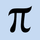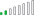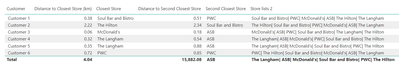cancel
Showing results for
Did you mean:Frequent Visitor

## Find the second and third nearest location based on Lat & Long

Hi,

I have two tables in my PowerBI model - where:

• Table 1 - lists individual Post Codes with their Lat and Longs (~390k post codes)
• Table 2 - lists individual Schools with their unique Post Codes and Lat and Longs (~2.5k schools)

I would like to add three new columns to Table 1 - where, for each post code, each column shows the top, second and third nearest school name based on the Lat & Longs.

I was able to calculate the nearest school for each post code by applying the "Closest Store" function provided in this solution by another community member (@OwenAuger , @PA_2022 , Find the nearest location for a customer )

I then modified the proposed solution to change "Firstnonblank" to "Lastnonblank" and set TopN to "2" and "3", respectively, in order to calculate the 2nd and 3rd nearest school to each postcode.

However, unfortunately the TopN function didn't always give me the right answer. For instance, when I requested the top 3 rows by Distance and set the order as "ASC", although the function returned the three closest schools to the selected Postcode, it did not sort those three rows in any particular order. So the "Lastnonblank" function returned the correct answer only one-third of the time.

Is there anyway I can further modify the formula to give me the right answer or do I need to think about this in a different way?

Any help would be greatly appreciated.

Many thanks,

ErdCan

2 ACCEPTED SOLUTIONSResolver II

Hi @erdcan , just seen that issue in the documentation for TopN - how fustrating!

I've taken a completely different approach:

`Store lists 2 = FIRSTNONBLANK(Stores[Store],if([Distance to Cust]=  maxx(topn(2,Stores,[Distance to Cust],asc),[Distance to Cust]),1,blank())) `
FirstNonBlank() - will take the first non-blank value in list.
The first parameter = value that you want to return
Second parameter = the expresion that will be compared for whether it is blank or not.

So what I have done, is asked PowerBI to return the first store, where an expression is not blank. And I have used an if statement to return "1" where the distance = maximum topn distance, and blank otherwise

So it's a bit of a hack, but seems to work?

Let me know!

PiResolver II

Checking against all options...

`Store lists 2 = FIRSTNONBLANK(Stores[Store],if([Distance to Cust]=  maxx(topn(1,Stores,[Distance to Cust],asc),[Distance to Cust]),1,blank()))&"|" & FIRSTNONBLANK(Stores[Store],if([Distance to Cust]=  maxx(topn(2,Stores,[Distance to Cust],asc),[Distance to Cust]),1,blank()))&"|" & FIRSTNONBLANK(Stores[Store],if([Distance to Cust]=  maxx(topn(3,Stores,[Distance to Cust],asc),[Distance to Cust]),1,blank()))&"|" & FIRSTNONBLANK(Stores[Store],if([Distance to Cust]=  maxx(topn(4,Stores,[Distance to Cust],asc),[Distance to Cust]),1,blank()))&"|" & FIRSTNONBLANK(Stores[Store],if([Distance to Cust]=  maxx(topn(5,Stores,[Distance to Cust],asc),[Distance to Cust]),1,blank()))&"|" & FIRSTNONBLANK(Stores[Store],if([Distance to Cust]=  maxx(topn(6,Stores,[Distance to Cust],asc),[Distance to Cust]),1,blank())) `

results in6 REPLIES 6Frequent Visitor

Hi @PiEye ,

Once again, many thanks for working on this.

I have now gone over your solution.

Writing the formulas as measures does make things a lot easier. Also, the "Distance to Second Closest Store" formula works really well and can easily be applied to figure out the distances to the 3rd and 4th, etc. stores.

However, I do think there is still an issue with the "Second Closest Store" formula. When I applied it to my dataset and substituted the "2" with "3" or "4" as you suggested, I still got the incorrect school names in some instances.

If you look at your screenshot as well, you will notice that for Customer 6 you get PWC as both your Closest Store as well as your Second Closest Store, which I believe is an error.

I think this is still due to the same problem with the TopN function, where when you ask it to order by [Distance to Cust] it does give you the closest 3 stores, but it doesn't sort the table, so the 2nd and 3rd store names do not always match the corresponding distance calculations to the 2nd and 3rd stores.

Hope this makes sense. Let me know if I've missed something please.

Many thanks,

ErdCanResolver II

Hi @erdcan , just seen that issue in the documentation for TopN - how fustrating!

I've taken a completely different approach:

`Store lists 2 = FIRSTNONBLANK(Stores[Store],if([Distance to Cust]=  maxx(topn(2,Stores,[Distance to Cust],asc),[Distance to Cust]),1,blank())) `
FirstNonBlank() - will take the first non-blank value in list.
The first parameter = value that you want to return
Second parameter = the expresion that will be compared for whether it is blank or not.

So what I have done, is asked PowerBI to return the first store, where an expression is not blank. And I have used an if statement to return "1" where the distance = maximum topn distance, and blank otherwise

So it's a bit of a hack, but seems to work?

Let me know!

PiResolver II

Checking against all options...

`Store lists 2 = FIRSTNONBLANK(Stores[Store],if([Distance to Cust]=  maxx(topn(1,Stores,[Distance to Cust],asc),[Distance to Cust]),1,blank()))&"|" & FIRSTNONBLANK(Stores[Store],if([Distance to Cust]=  maxx(topn(2,Stores,[Distance to Cust],asc),[Distance to Cust]),1,blank()))&"|" & FIRSTNONBLANK(Stores[Store],if([Distance to Cust]=  maxx(topn(3,Stores,[Distance to Cust],asc),[Distance to Cust]),1,blank()))&"|" & FIRSTNONBLANK(Stores[Store],if([Distance to Cust]=  maxx(topn(4,Stores,[Distance to Cust],asc),[Distance to Cust]),1,blank()))&"|" & FIRSTNONBLANK(Stores[Store],if([Distance to Cust]=  maxx(topn(5,Stores,[Distance to Cust],asc),[Distance to Cust]),1,blank()))&"|" & FIRSTNONBLANK(Stores[Store],if([Distance to Cust]=  maxx(topn(6,Stores,[Distance to Cust],asc),[Distance to Cust]),1,blank())) `

results inFrequent Visitor

Beatifully done, just genius 🙂 Thanks so much!Frequent Visitor

Hi @PiEye - thanks so much for putting time in this. I just got back from my break - let me have a play and come back to you.Resolver II

Hi @erdcan

Firstly, thank you for a well explained problem and example file - this helps a lot trying to find a solution!

I was able to solve the problem by using TOPN function and combining with either max or first non blank, and by using measures rather than calculated columns which makes it a bit easier to debug & sensitive to selections- but are easily translated to calculated columns if needed

Distance from customer

This is a measure in the "Stores" table that calculates the distance from customer. At a customer level I can now just drop the measure in rather than putting the whole calculation.

Distance to Cust =
VAR Lat1 = SELECTEDVALUE(Customers[Latitude])
VAR Lng1 = SELECTEDVALUE(Customers[Longitude])
VAR P =
DIVIDE ( PI (), 180 )
RETURN
MINX (
Stores,
VAR Lat2 = Stores[Latitude]
VAR Lng2 = Stores[Longitude]
//---- Algorithm here -----
VAR A =
0.5 - COS ( ( Lat2 - Lat1 ) * P ) / 2
+ COS ( Lat1 * P ) * COS ( lat2 * P ) * ( 1 - COS ( ( Lng2 - Lng1 ) * P ) ) / 2
VAR final =
12742 * ASIN ( ( SQRT ( A ) ) )
RETURN
final
).... This of course only works when one customer is selected, but is not of consequence as we are using it to form part of customer-context based calculations.

Nth nearest store

These expressions take advantage of TopN() in dax to return a table sorted by the Distance to the customer. Because it's at a customer level, each row returns it's "own" table if you get what I mean. I then take the max, because the max of the top 2 smallest is the 2nd smallest. "2" can be substituted for any number to get the 3rd, 4th, 5th etc

Distance to Second Closest Store = maxx(topn(2,Stores,[Distance to Cust],asc),[Distance to Cust])

Second Closest Store =
CALCULATE( FIRSTNONBLANK(Stores[Store],[Distance to Cust]),
topn(2,Stores,[Distance to Cust],asc))HTH

Pi

PS.. I have just started a blog, please check it out! https://www.iheartdemography.com/